# 推荐系统遇上深度学习(三)--DeepFM模型理论和实践

1、背景

DNN的局限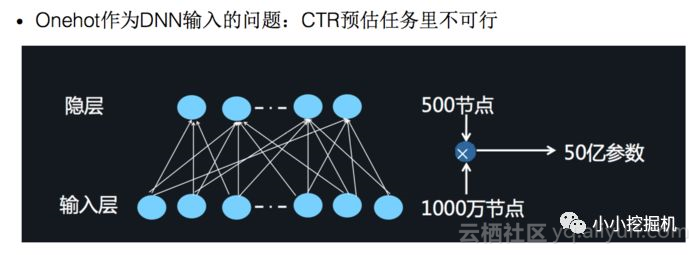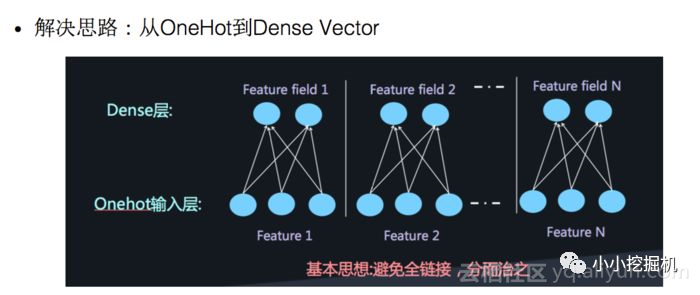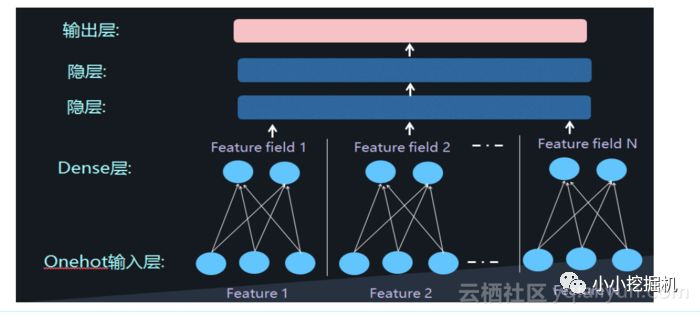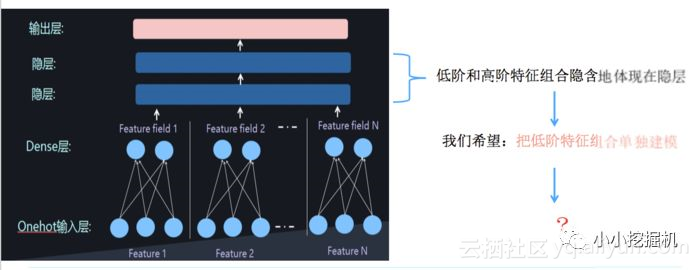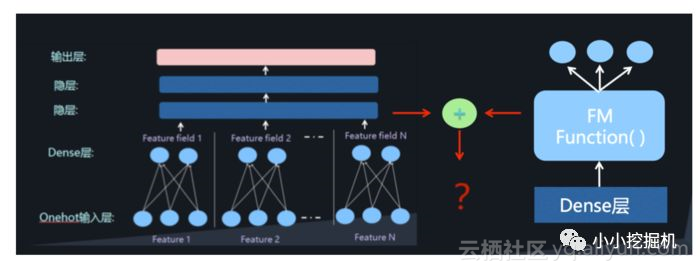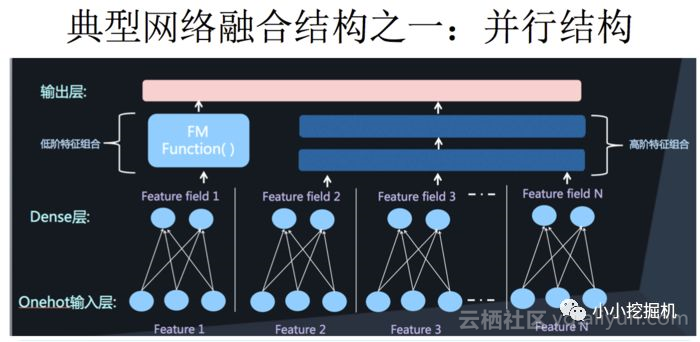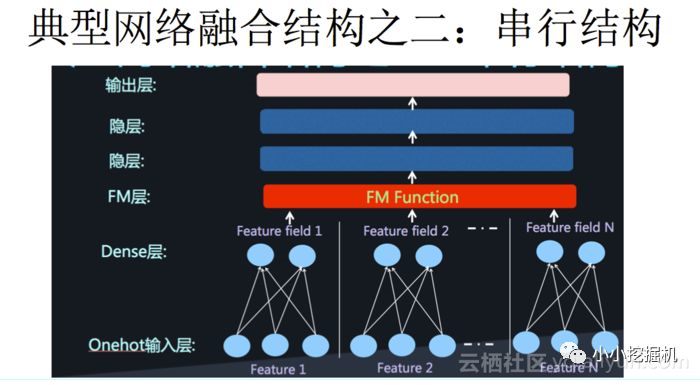2、DeepFM模型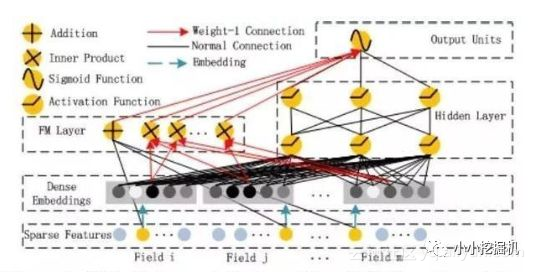DeepFM包含两部分：神经网络部分与因子分解机部分，分别负责低阶特征的提取和高阶特征的提取。这两部分共享同样的输入。DeepFM的预测结果可以写为：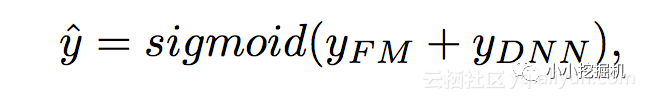FM部分

FM部分的详细结构如下：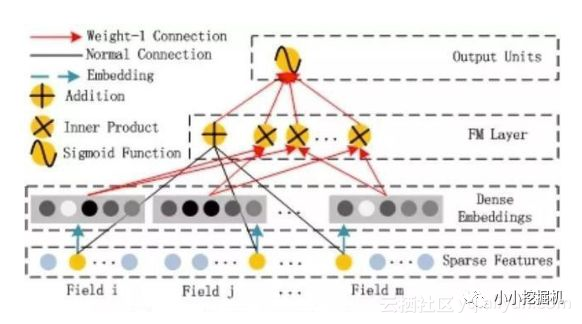FM部分是一个因子分解机。关于因子分解机可以参阅文章[Rendle, 2010] Steffen Rendle. Factorization machines. In ICDM, 2010.。因为引入了隐变量的原因，对于几乎不出现或者很少出现的隐变量，FM也可以很好的学习。

FM的输出公式为：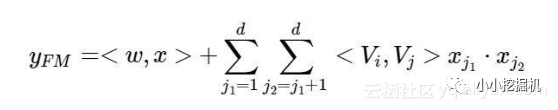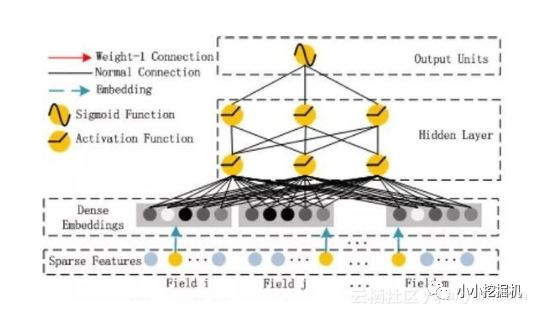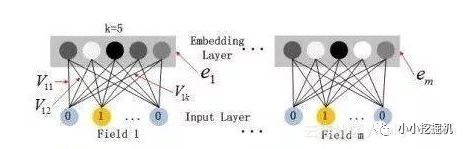3、相关知识

Gini Normalization

predictions = [0.9, 0.3, 0.8, 0.75, 0.65, 0.6, 0.78, 0.7, 0.05, 0.4, 0.4, 0.05, 0.5, 0.1, 0.1]
actual = [1, 1, 1, 1, 1, 1, 0, 0, 0, 0, 0, 0, 0, 0, 0]


Sorted Actual Values [0, 0, 0, 0, 1, 0, 0, 0, 1, 1, 0, 1, 0, 1, 1]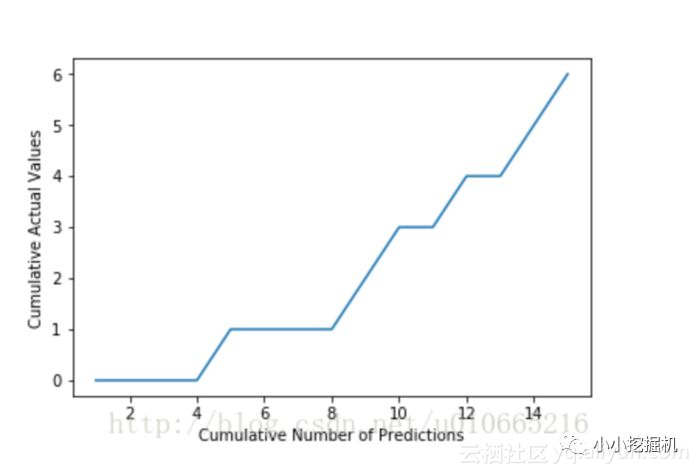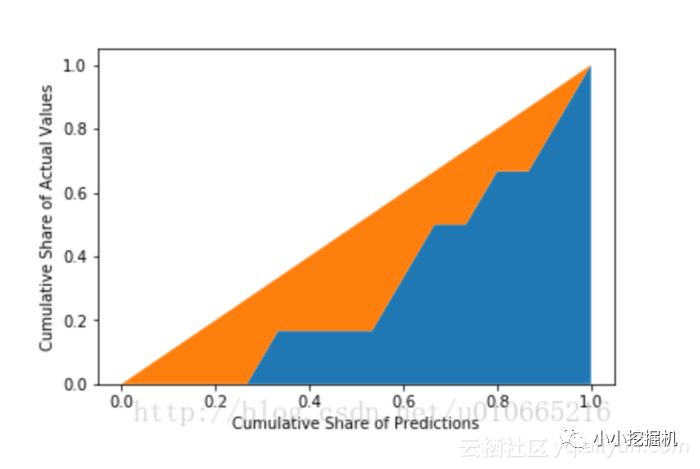embedding_lookup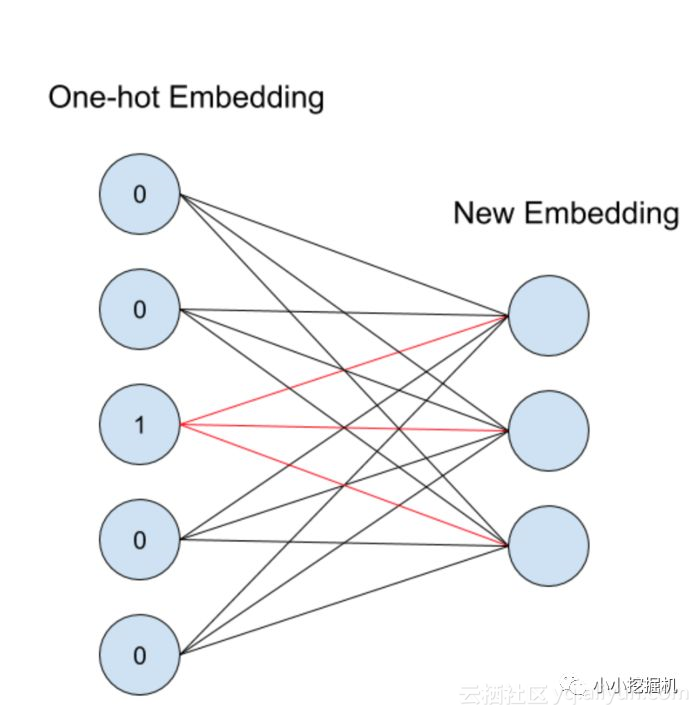4、代码解析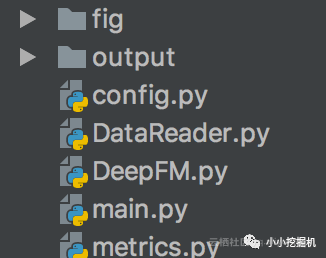self.feat_index = tf.placeholder(tf.int32,
shape=[None,None],
name='feat_index')
self.feat_value = tf.placeholder(tf.float32,
shape=[None,None],
name='feat_value')

self.label = tf.placeholder(tf.float32,shape=[None,1],name='label')
self.dropout_keep_fm = tf.placeholder(tf.float32,shape=[None],name='dropout_keep_fm')
self.dropout_keep_deep = tf.placeholder(tf.float32,shape=[None],name='dropout_deep_deep')


feat_index是特征的一个序号，主要用于通过embedding_lookup选择我们的embedding。feat_value是对应的特征值，如果是离散特征的话，就是1，如果不是离散特征的话，就保留原来的特征值。label是实际值。还定义了两个dropout来防止过拟合。

#embeddings
weights['feature_embeddings'] = tf.Variable(
tf.random_normal([self.feature_size,self.embedding_size],0.0,0.01),
name='feature_embeddings')
weights['feature_bias'] = tf.Variable(tf.random_normal([self.feature_size,1],0.0,1.0),name='feature_bias')


weights['feature_embeddings'] 存放的每一个值其实就是FM中的vik，所以它是N F K的。其中N代表数据量的大小，F代表feture的大小(将离散特征转换成one-hot之后的特征总量),K代表dense vector的大小。

weights['feature_bias']是FM中的一次项的权重。

Embedding part

# model
self.embeddings = tf.nn.embedding_lookup(self.weights['feature_embeddings'],self.feat_index) # N * F * K
feat_value = tf.reshape(self.feat_value,shape=[-1,self.field_size,1])
self.embeddings = tf.multiply(self.embeddings,feat_value)


FM part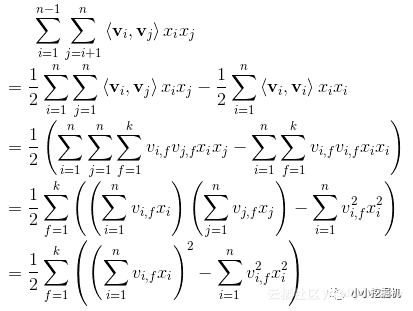# first order term
self.y_first_order = tf.nn.embedding_lookup(self.weights['feature_bias'],self.feat_index)
self.y_first_order = tf.reduce_sum(tf.multiply(self.y_first_order,feat_value),2)
self.y_first_order = tf.nn.dropout(self.y_first_order,self.dropout_keep_fm)

# second order term
# sum-square-part
self.summed_features_emb = tf.reduce_sum(self.embeddings,1) # None * k
self.summed_features_emb_square = tf.square(self.summed_features_emb) # None * K

# squre-sum-part
self.squared_features_emb = tf.square(self.embeddings)
self.squared_sum_features_emb = tf.reduce_sum(self.squared_features_emb, 1)  # None * K

#second order
self.y_second_order = 0.5 * tf.subtract(self.summed_features_emb_square,self.squared_sum_features_emb)
self.y_second_order = tf.nn.dropout(self.y_second_order,self.dropout_keep_fm)


DNN part

DNNpart的话，就是将Embedding part的输出再经过几层全链接层：

# Deep component
self.y_deep = tf.reshape(self.embeddings,shape=[-1,self.field_size * self.embedding_size])
self.y_deep = tf.nn.dropout(self.y_deep,self.dropout_keep_deep)

for i in range(0,len(self.deep_layers)):
self.y_deep = self.deep_layers_activation(self.y_deep)
self.y_deep = tf.nn.dropout(self.y_deep,self.dropout_keep_deep[i+1])


concat_input = tf.concat([self.y_first_order, self.y_second_order, self.y_deep], axis=1)


# loss
if self.loss_type == "logloss":
self.out = tf.nn.sigmoid(self.out)
self.loss = tf.losses.log_loss(self.label, self.out)
elif self.loss_type == "mse":
self.loss = tf.nn.l2_loss(tf.subtract(self.label, self.out))
# l2 regularization on weights
if self.l2_reg > 0:
self.loss += tf.contrib.layers.l2_regularizer(
self.l2_reg)(self.weights["concat_projection"])
if self.use_deep:
for i in range(len(self.deep_layers)):
self.loss += tf.contrib.layers.l2_regularizer(
self.l2_reg)(self.weights["layer_%d" % I])

epsilon=1e-8).minimize(self.loss)
initial_accumulator_value=1e-8).minimize(self.loss)
elif self.optimizer_type == "gd":
elif self.optimizer_type == "momentum":
self.optimizer = tf.train.MomentumOptimizer(learning_rate=self.learning_rate, momentum=0.95).minimize(
self.loss)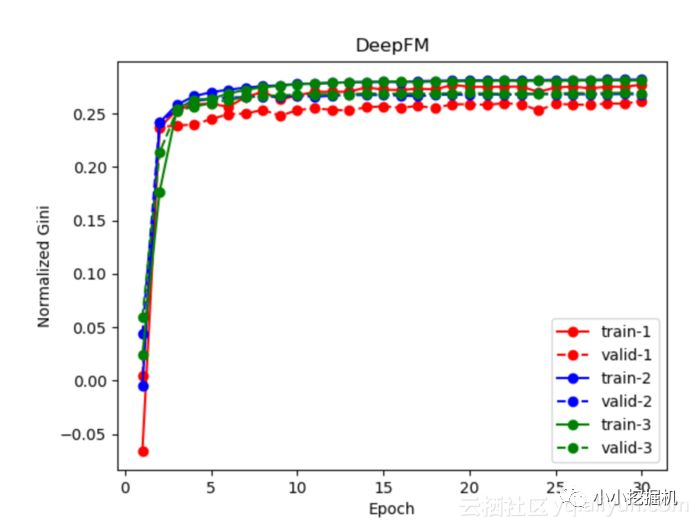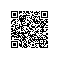Python爱好者使用钉钉扫一扫加入圈子
+ 订阅